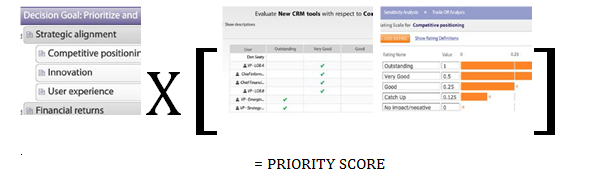# How Are Each Alternative's Scores Calculated?

The Ratings algorithms dramatically simplify the evaluation process by combining many metrics across multiple scales of measurement (called non-commensurate scales), enabling the organization to evaluate projects, programs, or products across a widely varied set of considerations. It then synthesizes them down into a quantified, single value rating number for comparison and sensitivity analysis purposes. Decision Lens ratings scores for the alternatives are acquired by multiplying scores on individual criteria by the weights assigned to those criteria, and then summing the total.

Ratings on individual criteria are placed on a 0 to 1 scale by using rating scales. In a verbal scale, a given verbal rating corresponds to a score between 0 and 1; that numeric score then enters the calculation. With a numeric scale, a user can input as many pairs of a numeric score and the corresponding score between 0 and 1 as is desired; for a given input, the score will be based on the linear relationship of those pairs.For example, consider a scale in which a score of 50 receives a 0 and a score of 100 receives a 1. To determine which DL score to assign to a 75, find 75 on the line between the points (50,0) and (100,1). That occurs at (75, 0.5), so the DL score is 0.5 CRITERIA WEIGHT X [AVERAGE OF RATINGS APPLIED TO RATINGS SCALE] X [ ] = PRIORITY SCORE. For the overall score calculation, consider a simple example. Suppose there are 3 criteria, with weights of 50%, 30%, and 20% respectively. An alternative receives grades that correspond on the 0-1 scale to 1, 0.25, and 0.5 respectively. Therefore, this alternative’s total score will be .50*1 + .30*.25 + .20*.5, or .675. A score cannot be higher than 1 or lower than 0.